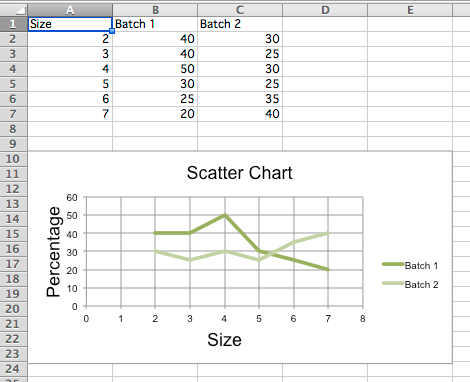# Scatter Charts¶

Scatter, or xy, charts are similar to some line charts. The main difference is that one series of values is plotted against another. This is useful where values are unordered.

```from openpyxl import Workbook
from openpyxl.chart import (
ScatterChart,
Reference,
Series,
)

wb = Workbook()
ws = wb.active

rows = [
['Size', 'Batch 1', 'Batch 2'],
[2, 40, 30],
[3, 40, 25],
[4, 50, 30],
[5, 30, 25],
[6, 25, 35],
[7, 20, 40],
]

for row in rows:
ws.append(row)

chart = ScatterChart()
chart.title = "Scatter Chart"
chart.style = 13
chart.x_axis.title = 'Size'
chart.y_axis.title = 'Percentage'

xvalues = Reference(ws, min_col=1, min_row=2, max_row=7)
for i in range(2, 4):
values = Reference(ws, min_col=i, min_row=1, max_row=7)
series = Series(values, xvalues, title_from_data=True)
chart.series.append(series)